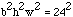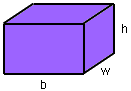Date: Mon, 27 Jul 1998 17:25:09 -0600 (CST)
Subject: Geometry

Name: James

Level: Secondary

Question:
I am going into 12th grade and am practicing for the SAT II. I have come across a problem that I cannot solve. It states that the front, side , and bottom faces of a retangular solid have areas of 24 square centimeters, 8 square centimeters, and 3 square centimeters, respectively. What is the volume of the solid. I need to know how to solve similar problems so is there a formula to go from area to volume?

Hi James,
To answer your last question 1st, there is no easy way to go from area to volume.
 For your first question, as in many geometry problems it helps to draw a labeled diagram and record the facts you know. Let's call the height h, the width w and the length b. Each of the faces of the solid is a rectangle and so we know that: bh = 24, hw = 8 and wb = 3 There are a number of ways to solve these three equations. One is to multiply the three equations to getwhich leads to bhw = 24 since b, h, and w are all positive. Since bhw = 24 and hw = 24 then b must be 1. Now you can easily find h and w and thus the volume of the retangular solid.I hope that this helps.
Jack

Go to Math Central